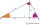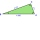# Right triangle calculator (a,b)

Please enter two properties of the right triangle

Use symbols: a, b, c, A, B, h, T, p, r, R

You have entered cathetus a and cathetus b.

### Right scalene triangle.

Sides: a = 110   b = 420   c = 434.1665866922

Area: T = 23100
Perimeter: p = 964.1665866922
Semiperimeter: s = 482.0832933461

Angle ∠ A = α = 14.67663931375° = 14°40'35″ = 0.25661513826 rad
Angle ∠ B = β = 75.32436068625° = 75°19'25″ = 1.31546449442 rad
Angle ∠ C = γ = 90° = 1.57107963268 rad

Height: ha = 420
Height: hb = 110
Height: hc = 106.4110944572

Median: ma = 423.5865882673
Median: mb = 237.0655391823
Median: mc = 217.0832933461

Vertex coordinates: A[434.1665866922; 0] B[0; 0] C[27.87695331021; 106.4110944572]
Centroid: CG[154.0121800008; 35.47703148573]
Coordinates of the circumscribed circle: U[217.0832933461; -0]
Coordinates of the inscribed circle: I[62.08329334609; 47.91770665391]

Exterior (or external, outer) angles of the triangle:
∠ A' = α' = 165.3243606863° = 165°19'25″ = 0.25661513826 rad
∠ B' = β' = 104.6766393137° = 104°40'35″ = 1.31546449442 rad
∠ C' = γ' = 90° = 1.57107963268 rad

# How did we calculate this triangle?

The calculation of the triangle progress in two phases. The first phase is such that we try to calculate all three sides of the triangle from the input parameters. The first phase is different for the different triangles query entered. The second phase is the calculation of other characteristics of the triangle, such as angles, area, perimeter, heights, the center of gravity, circle radii, etc. Some input data also results in two to three correct triangle solutions (e.g., if the specified triangle area and two sides - typically resulting in both acute and obtuse) triangle).

### 2. From cathetus a and cathetus b we calculate hypotenuse c - Pythagorean theorem:

Now we know the lengths of all three sides of the triangle, and the triangle is uniquely determined. Next, we calculate another its characteristics - same procedure as calculation of the triangle from the known three sides SSS.

### 4. Semiperimeter of the triangle

The semiperimeter of the triangle is half its perimeter. The semiperimeter frequently appears in formulas for triangles that it is given a separate name. By the triangle inequality, the longest side length of a triangle is less than the semiperimeter.

### 7. Calculation of the inner angles of the triangle - basic use of sine function

An incircle of a triangle is a circle which is tangent to each side. An incircle center is called incenter and has a radius named inradius. All triangles have an incenter, and it always lies inside the triangle. The incenter is the intersection of the three angle bisectors. The product of the inradius and semiperimeter (half the perimeter) of a triangle is its area.

The circumcircle of a triangle is a circle that passes through all of the triangle's vertices, and the circumradius of a triangle is the radius of the triangle's circumcircle. Circumcenter (center of circumcircle) is the point where the perpendicular bisectors of a triangle intersect.

### 10. Calculation of medians

A median of a triangle is a line segment joining a vertex to the midpoint of the opposite side. Every triangle has three medians, and they all intersect each other at the triangle's centroid. The centroid divides each median into parts in the ratio 2:1, with the centroid being twice as close to the midpoint of a side as it is to the opposite vertex. We use Apollonius's theorem to calculate the length of a median from the lengths of its side.

The right triangle calculators compute angles, sides (adjacent, opposite, hypotenuse) and area of any right-angled triangle and use it in the real world. Two independent properties entirely determine any right-angled triangle. The calculator provides a step-by-step explanation for each calculation.

A right triangle is a kind of triangle that has one angle that measures C=90°. In a Right triangle, the side c that is opposite of the C=90° angle, is the longest side of the triangle and is called the hypotenuse. The variables a, b are the lengths of the shorter sides, also called legs or arms. Variables for angles are A, B, or α (alpha) and β (beta). Variable h refers to the altitude(height) of the triangle, which is the length from the vertex C to the hypotenuse of the triangle.

Examples for right triangle calculation:

## A right triangle in word problems in mathematics:

• Triangle P2Can a triangle have two right angles?
• Height 2Calculate the height of the equilateral triangle with side 38.
• Vector 7Given vector OA(12,16) and vector OB(4,1). Find vector AB and vector |A|.
• Height of right RTThe right triangle ABC has a hypotenuse c 9 cm long and a part of the hypotenuse cb = 3 cm. How long is the height of this right triangle?
• HeightIs right that in any right triangle height is less or equal half of the hypotenuse?
• CablewayCableway has a length of 1800 m. The horizontal distance between the upper and lower cable car station is 1600 m. Calculate how much meters altitude is higher upper station than the base station.
• Euclid2In right triangle ABC with right angle at C is given side a=27 and height v=12. Calculate the perimeter of the triangle.
• Area of RT 2Calculate the area of right triangle whose legs have a length 5.8 cm and 5.8 cm.
• CalculateCalculate the length of a side of the equilateral triangle with an area of 50cm2.
• BisectorsAs shown, in △ ABC, ∠C = 90°, AD bisects ∠BAC, DE⊥AB to E, BE = 2, BC = 6. Find the perimeter of triangle △ BDE.
• RT triangle and heightCalculate the remaining sides of the right triangle if we know side b = 4 cm long and height to side c h = 2.4 cm.
• Trapezoid - RRFind the area of the right angled trapezoid ABCD with the right angle at the A vertex; a = 3 dm b = 5 dm c = 6 dm d = 4 dmThe double ladder shoulders should be 3 meters long. What height will the upper top of the ladder reach if the lower ends are 1.8 meters apart?The ladder has a length 3.5 meters. He is leaning against the wall so that his bottom end is 2 meters away from the wall. Determine the height of the ladder.Calculate the absolute value of complex number -15-29i.